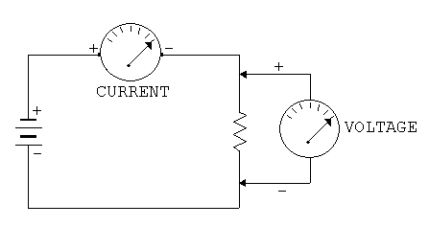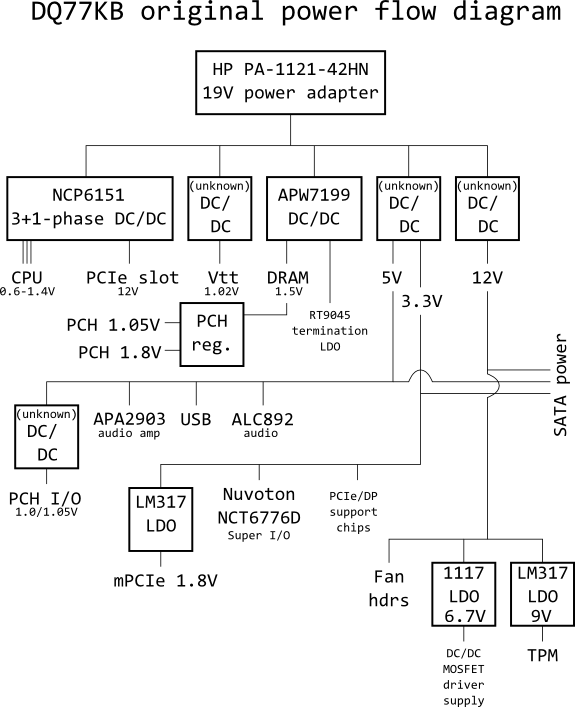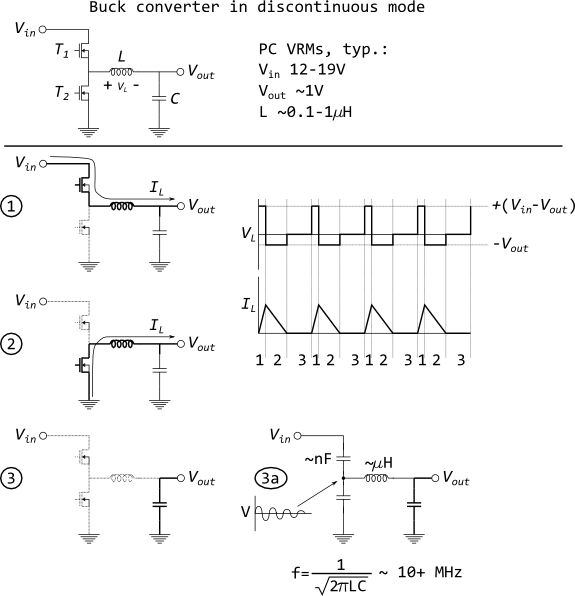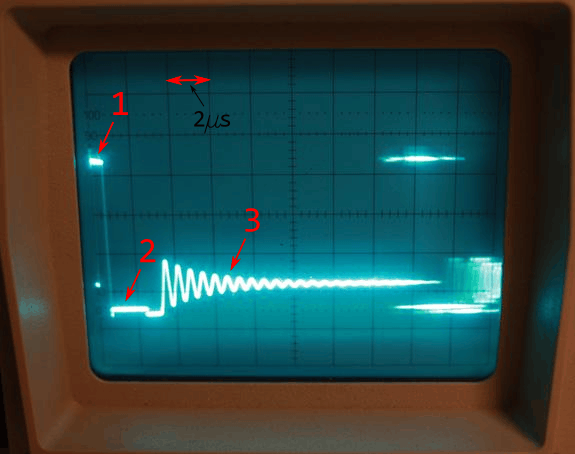## Techblog - Measuring current using VRM quirks

The past two weeks I have been busy writing a massive blog about Fluffy2, and how I got it to run on 5.9W. Today is the first part: an explanation on how to measure currents without cutting any tracks on a board.

I'm also on the twitters: @EfficientElec

#### How to measure power in a personal computer?

(Electrical) power consists of a voltage and a current. The most logical way to measure the power consumption of a circuit is to measure both the voltage and the electric current. To measure a voltage is very easy: You buy a multimeter, put one probe to a ground (one of the metal shields on the I/O ports of your computer, for instance) and the other one to the point you want to measure. To measure a current with a multimeter is a bit more complex: You would have to let the current run through your multimeter.This is of course fairly possible if the track is easily accessible. In Fluffy2, however, there are dozens of places where I would like to measure the electric current. Below is a diagram of the currents in the motherboard of Fluffy2, an Intel DQ77KB.Every line in this diagram is a place where I want to measure both the voltage and the electric current. Those lines are often difficult to get to: they might be inside the PCB, or too dangerous to cut, my multimeter might cause problems if I want to measure a current (see Burden Voltage). Besides, I only own 3 multimeters, so I can not measure multiple lines at the same time! In short: I need an alternative way to measure electric currents.
One method to do this, is to use a characteristic of DC-DC-converters (VRMs) in discontinuous mode. I will briefly explain how VRMs work, so you can understand what I mean.

###### How does a buck converter work?
Power is voltage times current. A current without a voltage, or a voltage without a current does not mean anything: Power is always both. Very roughly put, the voltage tells us the potential of the electricity, the current tells us 'how much' electricity runs through it. Most electrical devices need a very specific voltage to work: a European lightbulb needs 230V, a LED needs 3V and most chips in your computer need about 1V. Here in Europe, main power is 230V AC, so the electricity needs to be converted to a lower voltage. How does one do that?

With a power converter. Power conversion, DC-DC converters, electric power processing, power electronics, voltage regulation are all words for the same thing: To convert electric power from voltage A to a voltage B. There are two ways to do that, linear or switched. Today we will discuss the latter, a switched-mode converter and more specific: the buck converter. A buck converter converts a higher voltage to a lower voltage using an inductor.

If you do not know what an inductor is, you can imagine it as a bucket. The input voltage would be a tap and the output voltage a little stream. Or a river, depending on the size of your bucket. A switched-mode converter holds the bucket under the tap and empties it out into the river. How fast the bucket fills up depends on how fast the tap runs (input voltage). How fast it can empty the bucket depends on the stream and the amount of water it can handle. Too fast and the stream will overflow, too slow and the stream will dry up.

Mathematically an inductor will follow this rule:

v = L dI/dt

The voltage over an inductor is proportional to its inductance L (you can assume the inductivity of an inductor is constant) and the time derivative of the current. That means if you put a voltage over an inductor, the current will linearly increase with time. An inductor with an inductivity of 1 henry (H) with a potential difference of 1 volt (V) will experience the current going through it increasing at a rate of 1 ampère (A) per second. However, when you reverse the voltage - putting -1 volt over the inductor - the opposite happens and every second, the current will get 1 ampère smaller. This is all a switched-mode converter does: constantly putting a positive and subsequently a negative voltage over an inductor. Now we are ready for a picture:There is a lot going on in this picture, so let us focus on the figures (1), (2) and (3) first. These figures show what I have just tried to explain with (1) being the inductor with a positive voltage over it (input voltage on the left, output voltage on the right). A current will start to run through the inductor, as seen in segment 1 of the graph next to it. Subsequently, in (2), we tie the inductor to the ground on the left (input) side, thus imposing a negative voltage over it and letting the current decrease, as seen in segment 2 of the graph. Now for figure (3).

Figure (3) does not always happen. A buck converter usually runs continuously, or rather in continuous current mode (CCM). That is to say figures (1) and (2) would constantly alternate and the current through the inductor never decreases to zero. Figure (3) only happens if there is but a very light load on the output side. In this case, the converter needs to shut off every duty cycle, to prevent too much energy from being pumped into the output, thus increasing the output voltage. This is called the discontinuous current mode (DCM). In DCM, the converter does not do anything for the third part of the cycle.

###### Using DCM to measure energy
Now we are in interesting territory. DC-DC converters in discontinuous mode are great tools to measure energy. How? Let us go back to that figure with the number (3) and see how we got there.

In figure (1) we used the voltage difference between input and output to push energy into the inductor. Then, in figure (2), we extracted that energy from the inductor by imposing a negative voltage onto the inductor. And then, exactly when the inductor current hits zero amps, we shut off the entire converter. Well, I say exactly, but we all know that practice is never perfect. Pun slightly intended. These devices switch at a base frequency of around 1 MHz - that is: every switching cycle lasts only about 1µs. Even the most accurate timing will not be able to absolutely nail the timing. Consequently, the current through the inductor at the time of turn-off will not be exactly perfectly 0. There will be *some* energy still inside the inductor. But where can this energy go? There is no circuit anymore!

Well, there kind of is, there are parasitics. Those switches we used to tie the inductor to Vin and ground - they act like capacitors. And what do you get when you put a little bit of energy in a series capacitor-inductor circuit? Well, this type of circuit is very similar to the mechanical situation where you have a mass on a spring. If you put a little bit of energy (i.e. extend the spring) into a mass-spring system, it starts oscillating. And that is what an inductor-capacitor circuit does as well. The parasitic capacitance and inductance together cause oscillations in the order of tens of MHz.

This is something we can measure with an oscilloscope and use to our advantage. Here we go:This image tells us so much about the VRM at hand. For your information: the VRM we are looking at with this picture is an 18.8-to-3.19V DC-DC converter with a 7.3µH +/-10% inductor. So, what information do we get from this image?
• First of all, it is definitely a buck converter running in DCM. We can tell by the oscillation pattern (3) following the normal switching pattern (1) and (2).
• The second obvious metric is the PWM base frequency. This oscilloscope was set at 2µs/div, that means that every vertical line denotes 2µs of time. All the way on the leftmost denomination is the start of the cycle, and somewhere around the 8th line is the next cycle. By the way, the first cycle is very sharp while everything following it is fuzzy and hard to make out. This is because the frequency varies from cycle to cycle, and the oscilloscope does not quite follow. This changing-of-frequency is done because it reduces acoustic noise and electromagnetic interference. It is called 'spread spectrum'. Anyway, the total cycle lasts about 8 divisions, which is 16µs. That means the PWM frequency is about 60kHz.
• The duty cycle of the top switch, which is the proportion of time that the top switch is on. As you might be able to make out, this is about 0.55µs (a bit more than a quarter of the first division), so the duty cycle is the proportion of that to the entire cycle, which is 0.55/16=3.4%
• Likewise, the duty cycle of the bottom switch. This is about 2.2µs or 13.75%
Alright, so now we know for how long the inductor is charged, discharged and how often this repeats every second. We also know the value of the inductor. See what I am doing here? Now we have all the information we need to calculate the power delivered by this DC-DC converter! So... where to start.... Let us calculate the peak inductor current. We know that:

U = L dI/dt

Where during state 1, U=18.8-3.19V, L=7.3µH and dt=0.55µs. So we can calculate dI, which I calculated to be 1.176A. So during state 1 we put this much energy into the inductor:

Emag = (1/2) L I2 = 5.049µJ

This repeats 60,000 times per second, so this DC-DC converter magnetically exchanges 60000*5.049µJ=0.3J/S=0.3W of power. But this is not all the power it processes! We are missing the fact that during state 1, the input directly transfers some power to the output through the inductor current. We can calculate this to be:

Edirect = (1/2) IL,peak*Vout*t1

What I have done here is calculate the average current during state 1, and then multiplied it by the output voltage and the time that state takes. Like the magnetically transferred power, this repeats 60,000 times per second to yield Edirect = 0.062 W. Summing these two effects together gives us the total power converted by this DC-DC converter: Emag + Edirect=0.36W at an output voltage of 3.19V. Done!

#### Conclusion

This is how you measure - en masse - current in a complex power distribution without having to desolder parts to put an ammeter in. This might not have been the most exciting blog I have ever written, but this kind of information is very useful to those few people with an oscilloscope who would like to measure power flow in their devices, but do not feel like desoldering and messing up their precious hardware. And in trying to convey this technique, I also explained how buck converters work. A double win. See you next time!10-'12 Fluffy2 - stroommeting & hoe werkt een VRM?10-'12 MADPSU is jouw stem waard!

#### Reacties

Er zijn nog geen reacties op deze post

Om te kunnen reageren moet je ingelogd zijn. Via deze link kun je inloggen als je al geregistreerd bent. Indien je nog geen account hebt kun je er hier één aanmaken.